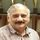cancel
Showing results for
Did you mean:Regular Visitor

## How do i calculate sum of three rows for each rows with different values

How do i calculate sum of three rows for each rows for exampple TableA(ColumnA+ColumnB+Column C) in New Calculated Column D i want to show the sum like given below? Please help!

I am getting the same values for all the rows? I want the result as Column D which is total of col a, b and c.

A  B  C      D
1  1  0       2 ( Sum of A+B+C as 1+1+0)
0  1  0       1
1  1  1       3

1 ACCEPTED SOLUTIONMicrosoft

@asta

Just try like below.

```Column D =
TableA[A] + TableA[B] + TableA[C]```Best Regards,

Herbert

5 REPLIES 5Microsoft

@asta

Just try like below.

```Column D =
TableA[A] + TableA[B] + TableA[C]```Best Regards,

HerbertRegular Visitor

Yeah that was pretty easy like this..it did work !Microsoft

@asta

If you have got your problem solved, you could mark the right answer as solution to close this thread.Best Regards,

HerbertCommunity Champion

hi @asta

In a Calculated Column:

SumABC = CALCULATE(SUMX(Tabla1,Tabla1[A]+Tabla1[B]+Tabla1[C]))

Lima - PeruCommunity Champion

Hi @asta

Try the folliwng using sumx to iterate over each row.

ColumnD=sumx(Yourtable, (cola+colb+colc))

If this works please accept this as solution and also give KUDOS.

Cheers

CheenuSing

Did I answer your question? Mark my post as a solution and also give KUDOS !

Proud to be a Datanaut!